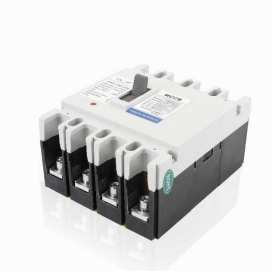# How does transformer be matched with circuit breaker?

How does transformer be matched with circuit breaker?

For example, the power transformer be 2000kVA, and the impedance voltage Uk=6%. The rated voltage of the low-voltage side winding is 400V/230V (line voltage/phase voltage).

Step 1: Calculate the rated current of the power transformer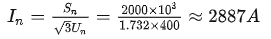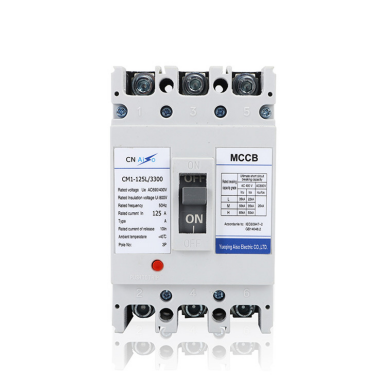Step 2: Calculate the short-circuit current of the power transformer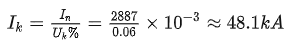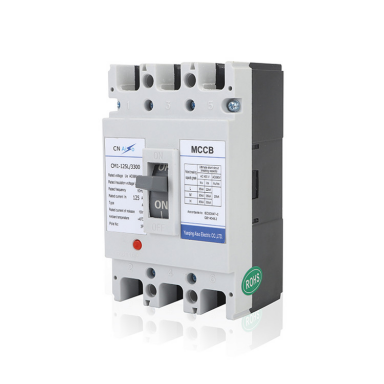Step 3: Optional circuit breaker

The rated current Ie of the circuit breaker must be greater than the rated current In of the power transformer, and the ultimate short-circuit breaking capacity Icu of the circuit breaker must be greater than the short-circuit current of the power transformer.